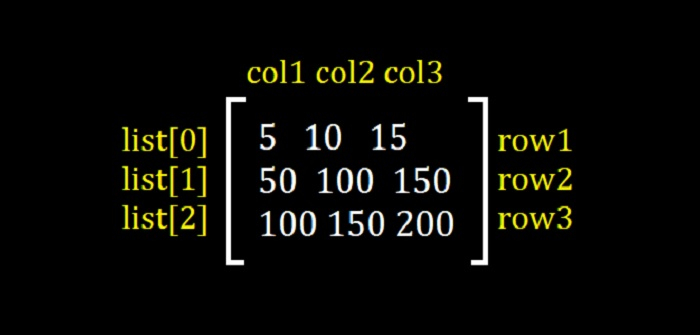# Numpy Array advantage over a Nested List

In this article, we will learn about the advantages of a Numpy array with a Nested List in Python. The Numpy array definitely has advantages over a Nested. Let’s see the reasons −

• The array in Numpy executes faster than a Nested List.
• A Nested List consumes more memory than a Nested List.

## Numpy Array

NumPy is an N-dimensional array type called ndarray. It describes the collection of items of the same type. Items in the collection can be accessed using a zero-based index.

Every item in an ndarray takes the same size of block in the memory. Each element in ndarray is an object of data-type object

## Create a Numpy Array

### Example

The basic Numpy Array is created using an array() function in NumPy:

import numpy as np

# Create a Numpy Array
arr = np.array([5, 10, 15, 20, 25])
print("Array = ",arr)


### Output

Array = [ 5 10 15 20 25]


## Create a Matrix with Numpy

### Output

In this example, we will create a matrix using the numpy library −

import numpy as np

# Create a Numpy Matrix 2x3
a = np.array([[5, 10, 15], [20, 25, 30]])

# Display the array with more than one dimension
print("Array = ",a)


### Output

Array =
[[ 5 10 15]
[20 25 30]]


## Nested Lists

A nested list as the name suggests is a list of lists. They can be used to create a matrix as well.

## Create a Matrix with Nested Lists

With Nested Lists, you can easily create a matrix in Python. In a Nested List.

• Every element of the nested list i.e., a matrix has rows and columns.
• Number of elements in the nested lists = The number of rows of the matrix.
• Length of the lists inside the nested list = number of columns.

### Example

Let us see an example −

# create a matrix 3x3
mat = [[5, 10, 15],
[50, 100, 150],
[100, 150, 200]]

# number of rows
rows = len(mat)
print("Number of rows = ", rows)

# number of columns = length of sublist
cols = len(mat)
print("Number of columns = ", cols)

# Display the matrix (nested list)
print("\nMatrix = ")
for i in range(0, rows):
print(mat[i])


### Output

Number of rows =  3
Number of columns =  3

Matrix =
[5, 10, 15]
[50, 100, 150]
[100, 150, 200]


The above example displays nested list −